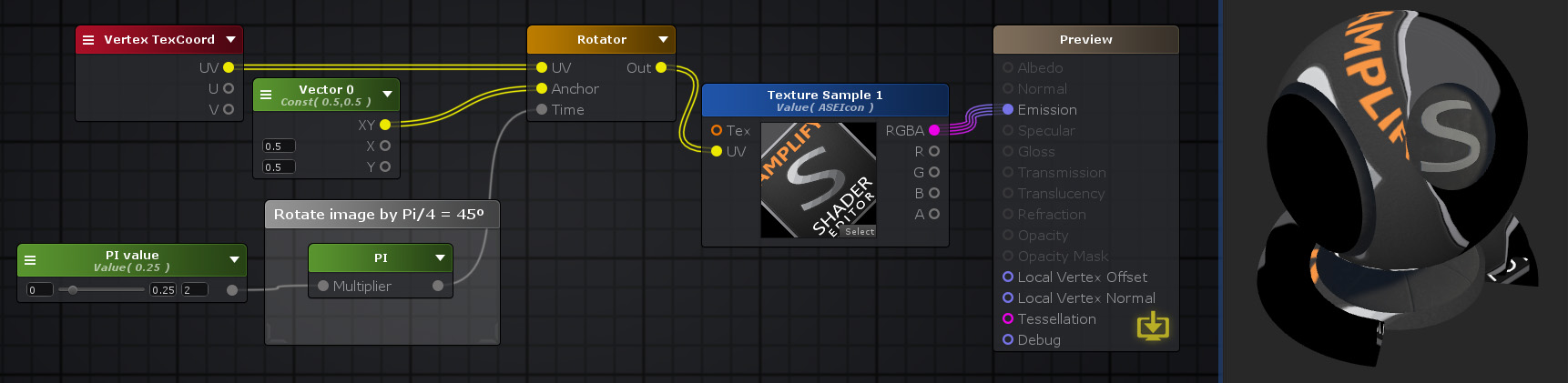## Pi Node

The Pi node outputs the mathematical constant π ( 3.14159265359... ) multiplied by a scalar factor defined by its Multiplier property.Nodes used: Vertex TexCoord, Vector2, Float, PI, Rotator, Texture Sample

Node Parameter Description Default Value
Multiplier Scalar factor which is multiplied by the π constant. Only visible if the respective input port is not connected. 0

Input Port Description Type
Multiplier Scalar factor which is multiplied by the π constant. Float

 Math Operators - PI